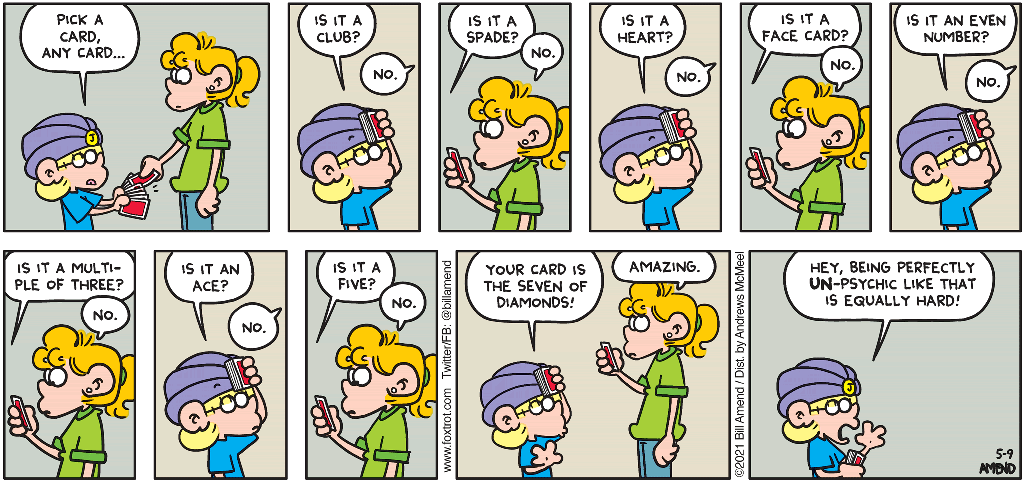``` Recursion My latest programming effort involves a card guessing game. I was motivated by my favorite cartoonist Bill Amend's latest strip (FoxTrot). The language I use most frequently (TinyC) does not support what is called "floating point" arithmetic. It supports "integer" arithmetic. I wanted to add a feature to un-psychic.tc that would show the average number of guesses it took to discover the "secret card" in the game. If it took 6 guesses in game #1 and 7 guesses in game #2 the average would be 6.5. This is a "floating point" number. A function I wrote called dpf (display proper fraction) was used to get around the integer arithmetic limitation of TinyC. Computer programs use what are called functions. Functions usually need to be fed what are called arguments. Functions are often housed in libraries (like books). Functions have names. For example the function dpf takes 3 arguments: numerator, denominator, precision. dpf 3, 7, 4 would cause the decimal number .4285 to display on a terminal because .4285 is the 4 decimal digit precision value of the fraction 3/7. If you play the card guessing game 7 times and it takes you a total of 38 guesses to identify the secret card your average number of guesses per game would be 38/7 which is 5.4285 (to 4 decimal digits precision). The whole number part of 38/7 is 5. The fractional part is 3/7. Here's the code for dpf // display proper fraction dpf int n, d, p [ if p > 0 [ pn 10 * n / d dpf 10 * n % d, d, p - 1 ] ] Notice how the definition of this function uses itself (thus it is recursive). If you manually try to figure out what 3/7 is by dividing 7 into 3 (remember long division?) you see that it involves multiplying the numerator by 10, putting the whole number part of the quotient up top and then repeating the process with the remainder part of the quotient. .4285 ________ 7 | 3.0000 -2 8 --- 20 -14 --- 60 -56 --- 40 -35 --- etc. The / operation gives you the whole number part of a division. The % operation gives you the remainder part of a division. Each "call" or "use" of the function tests the precision to see if it's still positive (if p>0) and if so, does its thing with new arguments (p is reduced by 1 each time so it'll eventually not be positive).Here is another example of recursion: minarr.tc The above translated to C and QB64: minarr.c minarr.bas ```